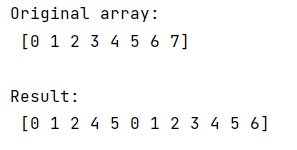# Index multiple, non-adjacent ranges in NumPy?

Learn, how to index multiple non adjacent ranges in Python NumPy?
Submitted by Pranit Sharma, on March 05, 2023

Suppose that we are given a numpy array and we need to select multiple and non-adjacent ranges from this array.

For example, if we are given an array as [1,2,3,4,5,6,7,8,9,10] and we need to select multiple ranges like [0:3] and [4:6] and [7:].

To index multiple, non-adjacent ranges, we need to concatenate, either before or after indexing. Numpy provides a good function called numpy.r_() which is used to concatenate multiple sliced values along the 1st axis.

Let us understand with the help of an example,

## Python code to index multiple, non-adjacent ranges in NumPy

```# Import numpy
import numpy as np

# Creating a numpy array
arr = np.array([0, 1, 2, 3,4, 5, 6, 7])

# Display Original array
print("Original array:\n",arr,"\n")

res = np.r_[0:3,4:6,7:]

# Display result
print("Result:\n",res,"\n")
```

Output:Languages: » C » C++ » C++ STL » Java » Data Structure » C#.Net » Android » Kotlin » SQL
Web Technologies: » PHP » Python » JavaScript » CSS » Ajax » Node.js » Web programming/HTML
Solved programs: » C » C++ » DS » Java » C#
Aptitude que. & ans.: » C » C++ » Java » DBMS
Interview que. & ans.: » C » Embedded C » Java » SEO » HR
CS Subjects: » CS Basics » O.S. » Networks » DBMS » Embedded Systems » Cloud Computing
» Machine learning » CS Organizations » Linux » DOS
More: » Articles » Puzzles » News/Updates